# Column vs. Row | All Definitions & Differences

Column vs. Row: In simple words, a row is a straight line of something. On the other hand, a column is an upright order of something. However, there are a lot of other meanings of the word ‘row’ and ‘column’. The use of rows and columns helps us in finding out differences and grouping certain information easily. In this article of “Column vs. Row,” we will learn about the differences between columns and rows for the following topic- ‘Column vs. Row Excel’, ‘Column vs. Row Matrix’, ‘Column vs. Row Periodic Table’ and much more.

## What are Rows?

A chain of data that is put down out horizontally in a table or worksheet is a Row. It is a horizontal grouping of objects, words, numbers, and data. We place data objects face-to-face with lying next to each other on the straight line in a Row.

## What are columns?

A column is a vertical chain of cells in a chart, table, or worksheet. We enter data is in an area on a worksheet known as a cell. It is an arrangement of numbers, facts, words, etc. We usually place a column one after another in an ongoing order. We mainly divide columns from each other by lines in a table. This helps to increase the ease of reading and presentation.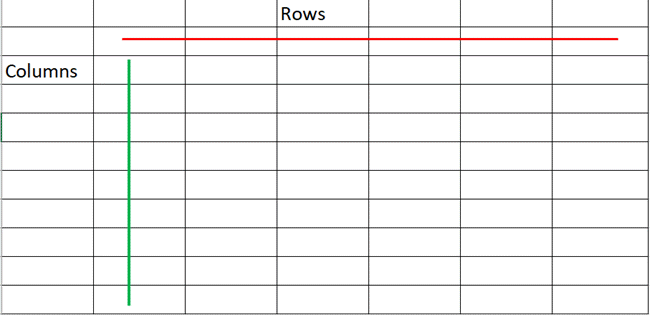### Column vs. Row MS Excel

Microsoft Excel shows data in a table-like form. We put out information in a table containing rows and columns. Rows and columns are two different things that jointly make up a table. These are the two most important points of Excel that allow users to store and use their data.

### Rows in Excel

We can see on the left-hand side that each and every row is known by a unique numeric value.We arrange the row numbers on the worksheet horizontally. It ranges from 1-1,048,576 (you can have a total of 1,048,576 rows in Excel). In the same way, the rows themselves run horizontally on a worksheet.  Likewise, in the table, we place the data horizontally. As a result, it goes across from left to right.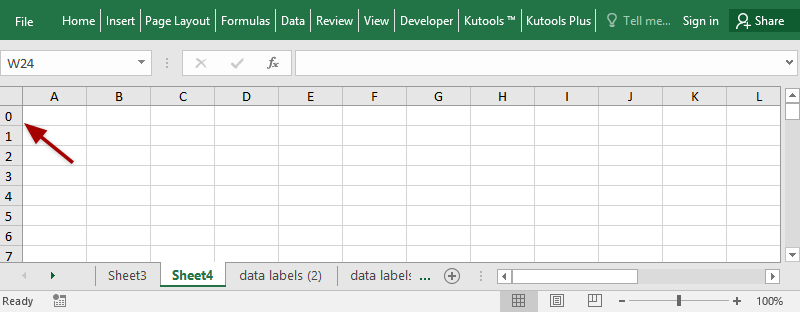### Columns in Excel

Columns stand for and are known by a unique alphabetical head letter, which is found at the top of the worksheet. Column head starts from A-XFD, as Excel spreadsheets can have 16,384 columns as a whole. Columns run vertically in the worksheet. As a result, the data goes from up to down. The first column in Excel is Column A.### Cell in Excel

A cell is the joining of a row and a column. Therefore, a row and a column next to each other makes a cell. We can define a cell by the mixing of a row number and a column header. For example, the selected cell is C3 in the image. It has a column head C and row number 3. We can also select a whole row or column from a cell. For selecting the whole row when in any cell, press Shift Space. For selecting the whole column when in any cell, press Ctrl-Space.

### Column vs. Row in Excel Differences

• Rows are the horizontal lines in the worksheet. On the other hand, columns are the vertical lines in the worksheet
• The total rows are 10, 48,576 in the worksheet. However, the total columns are 16,384.
• Rows range from 1 to 1,048,576 in the worksheet. At the same time, columns are ranging from A to XFD.
• To select an entire fixed row, press the Shift + Space bar. But, to select the entire column, press the Ctrl+ Space bar.
• The default row height is 18.75 pt. and 25 pixels. On the other hand, the default width of the column is 8.43 pt. and 64 pixels.
• We represent each row by a number. Whereas, we show each column by an alphabet.
• In a table, there are four main components: the caption, box-head, stub, and body. In a table, the top-most part, where columns are displayed, is called a caption. As against this stub, the left part of the table expresses the rows.

## Column vs. Row in Matrix

In mathematics, a matrix (plural matrices) is a rectangular table of numbers, symbols, or expressions. We lay out these numbers, symbols or expressions in rows and columns. It is used to show a mathematical object or a property of such an object.

## Rows in Matrix

A Row matrix is a rectangular order of elements, placed in a horizontal line. A row matrix is a type of matrix that has a single row. However, the number of columns could be more than one. For this reason, if the matrix is in the order of 1 x n, then it is a row matrix. The elements are arranged so that each row represents a row in the matrix. However, different from the row matrix, a column matrix will have a single column. A row matrix has only one row.

It has many columns. The number of elements in a row matrix is equal to the number of columns in the matrix. It is also a rectangular matrix. The transpose of a row matrix is a column matrix. A transpose of a matrix which we get by changing its rows into columns (and in the same way, its columns into rows).The row matrix is added or subtracted to only a row matrix of the same order. A row matrix is multiplied with a column matrix only. The product of a row matrix with a column matrix gives a single matrix.

### Columns in Matrix

A column matrix is a matrix that has all its elements in a single column. We lay out the elements vertically. The order of a column matrix is n x 1. A column matrix has only one column. It can have many rows, which are equal to the number of elements in the column. The order of a column matrix is n × 1. Therefore, it has n elements. A column matrix has only one column.

It has many rows. The number of elements in a column matrix is the same as the number of rows in the matrix. It is also a rectangular matrix. The transpose of a column matrix is a row matrix. We only perform additions or subtractions to column matrices in the same order. There is only one way to multiply a row matrix with a column matrix. The product of a column matrix with a row matrix gives a single matrix.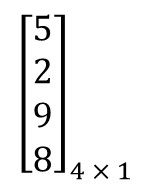### Column vs. Row in Matrix Differences

• Rows are horizontal arrangements. On the other hand, columns are the vertical arrangement.
• The order of a row matrix is 1 x n. The order of a column matrix is n x 1.
• The transpose of a row matrix is a column matrix. Whereas, column matrix transpose is a row matrix.

### Column vs. Row Periodic Table

A periodic is also known as the periodic table of chemical elements. It is a table like display of the chemical elements. For this reason, chemistry, physics, and other sciences use the periodic table widely. However, it is generally seen as an icon of chemistry. Here, it is a visual statement of the periodic law.  At the same time, it states that the properties of the chemical elements show a periodic dependence on their atomic numbers.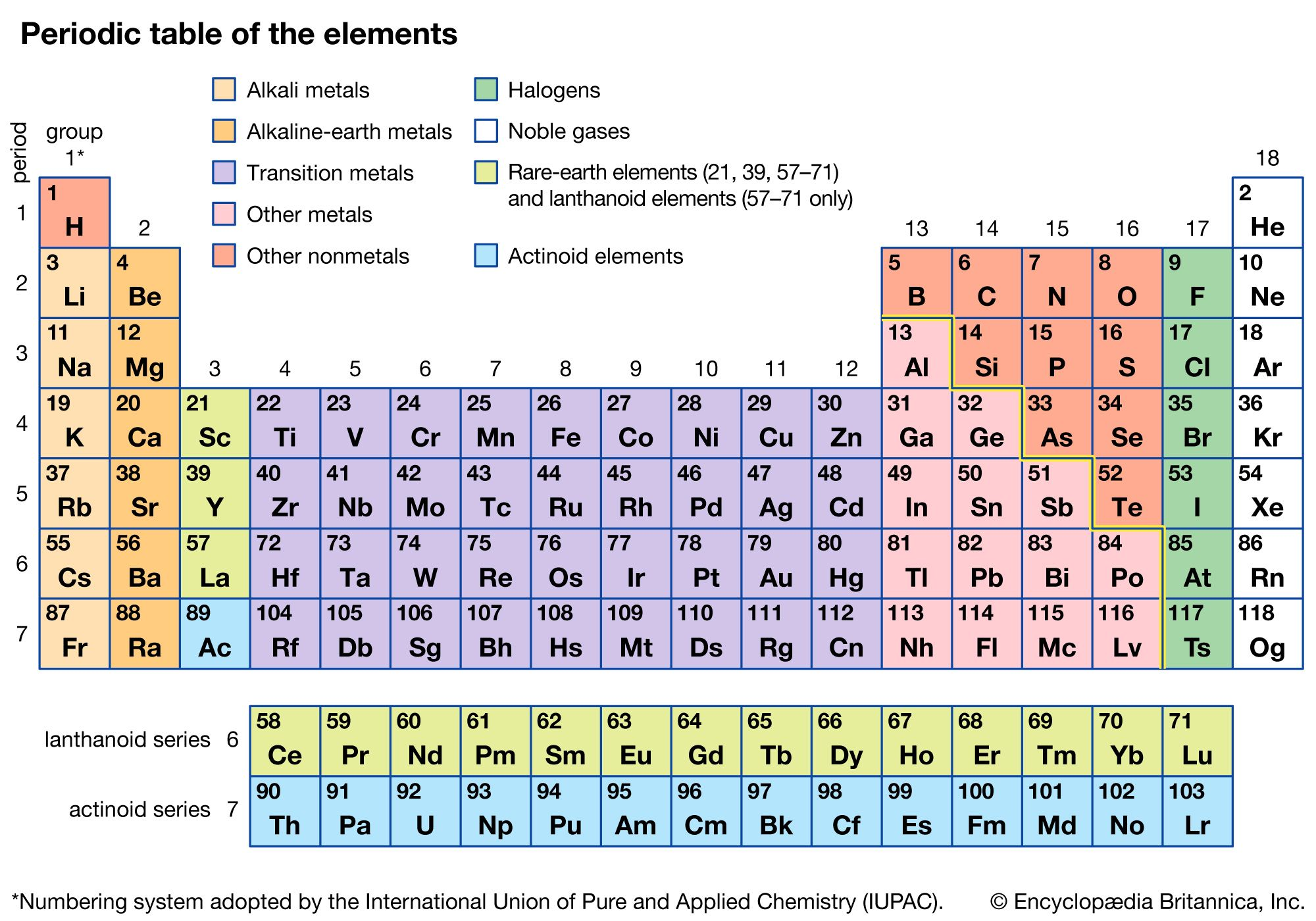## Rows in Periodic Table

A period is a row of chemical elements in the periodic table. We arrange the chemical elements in seven horizontal rows. This time, the inner transition metals are in the two rows at the bottom of the table.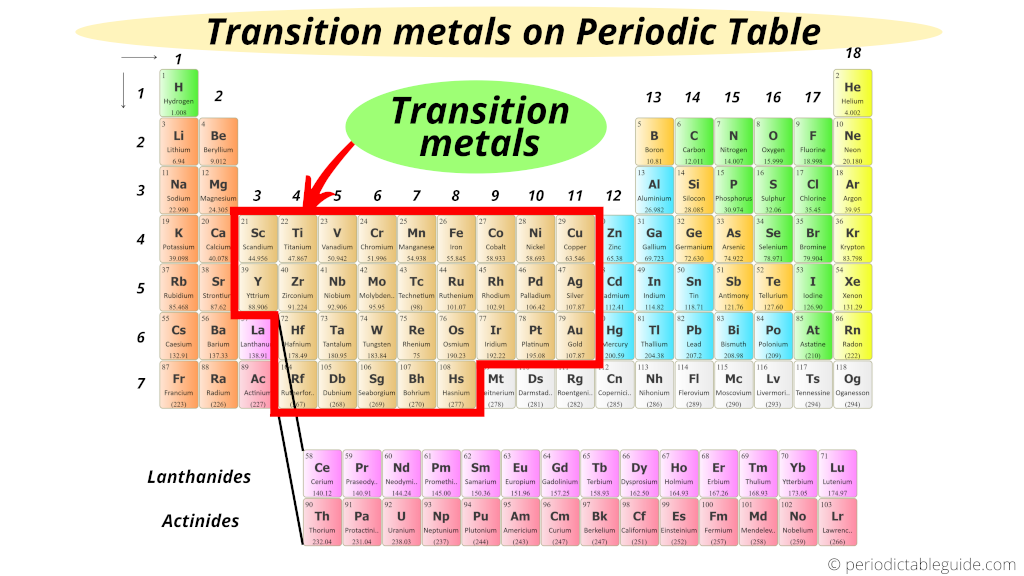### Columns in Periodic Table

Periodic tables are divided into groups or families by their vertical columns. We lay out the chemical elements in 18 vertical columns. Even more, the elements are also labeled into the main-group elements in columns numbering 1, 2, and 13–18. The transition metals in the columns are labeled 3–12.

### Column vs. Row Conclusion

Columns and rows give us a way to manage data properly. In Excel, rows, and columns make up a worksheet. Rows and columns make up a Google spreadsheet. It’s easy to add mathematical formulas to Excel columns and rows.

### Column vs. Row FAQs (Frequently Asked Questions)

#### 1. What is a row and column in Matrix?

Rows are the horizontal arrangement of numbers. On the other hand, columns are the vertical arrangement of data.

2. How do we arrange elements in rows and columns?

We arrange elements from left to right in rows. At the same time, we lay down elements from top to bottom in columns.

3. How are the headings of rows and columns represented in a spreadsheet?

In a spreadsheet, we represent headings of rows with numbers. On the other hand, we represent headings of columns with alphabets.

4. What do we call a column in a database table?

Tuples and rows are both examples of records. In the first place, a column in a table is a field. We can also know it as an attribute. We can also think of it like this. A record is also known as an attribute. It is a record that contains a set of attributes.

5. Does a row or column come first?

We refer to a matrix’s row and column measurements or order as its dimension. However, in the latest years, rows have taken place over columns.

6. Is it always a table with columns and rows?

A columnar table consists of rows and columns. In comparative databases and flat file databases, a table is a collection of data elements value with vertical columns. On the other hand, in horizontal rows, the cell represents the joining of the row and column.

#### 7. How do you find rows and columns?

To find rows and columns, check the types of order. On the other hand, if the order is vertical or lengthwise it is a column. Otherwise, it is a row.

8. Is a column vertical or horizontal?

In a table, a row is a horizontal row of data. On the other hand, the column is a vertical column of cells. A row runs across the top of the report. However, we order columns from top to bottom.

9. How do you spot rows and columns?

A row is the set of cells from left to right. On the other hand, a column is the set of cells from top to bottom.

10. What is the difference between cell and row?

The part of a row joined with a column forms a cell. Further, a row is a straight chain-like order of cells is placed horizontally.

How do I switch rows and columns in Google Sheets?

• Firstly, select the rows you want to change.
• Secondly, right-click. Next, select Copy.
• Further, select the cell in which you want to change the data.
• Furthermore, click-Right. Click on Paste Special. Then, Paste Transposed.
• Next, you will see now, the data has been changed.
• Finally, delete the previous or added data. Lastly, format the rows and columns according to the data size.

12. Is a vector a column or row?

Column vectors and row vectors are two different kinds of matrices. Wherever we draw a horizontal pattern it is a row vector. On the other hand, when we draw the vector is in a vertical form, it is a column vector.

#### 13.  Do the column store and row store tables kept in memory all the time?

At the time of startup, the row store loads the memory. For this reason, we can store it forever. For that reason, it is not possible to unload parts of the row store.

The column store table columns load the memory. It happens when they are gained or based on a reload algorithm. For this reason, we can unload the memory to use it for another purpose also. Further, they are explicitly unloaded or after a defined retention period.

14. What are the cons of column vs. row?

Rows and columns on a worksheet are not useful for writing paragraph content. The reason is, they are not rows or columns.

15. How to easily recognize a column vs. row?

A column goes up and down. On the other hand, a row goes side to side.